Get inspired by the success stories of our students in IIT JAM MS, ISI  MStat, CMI MSc DS.  Learn More

# ISI MStat 2019 PSA Problem 16 | Area bounded by the curveThis is a problem from ISI MStat 2019 PSA Problem 16 based on calculating area bounded by the curve.

## Area bounded by the curve - ISI MStat 2018 PSA Problem 16

The functions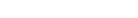are given by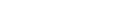and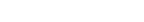What is the area enclosed between the graphs of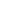and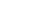• (A) 1/8
• (B) 1/4
• (C)5/12
• (D) 7/24

### Key Concepts

Integration

Graph of a function

ISI MStat 2019 PSA Problem 16

Introduction to Real Analysis by Bertle Sherbert

## Try with Hints

Inverse of a function is basically reflection about y=x line .

So , we can getand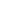from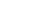and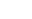respectively by replacing x by y and y by x .

Let's draw the curves .

This is graph of inverses of f and g when they are defined in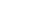. But in our problem we should consider one positive x axis and y axis .

Therefore area of the curve bounded by the graphs ofandis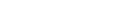= 1/8 .

## Subscribe to Cheenta at Youtube

This is a problem from ISI MStat 2019 PSA Problem 16 based on calculating area bounded by the curve.

## Area bounded by the curve - ISI MStat 2018 PSA Problem 16

The functionsare given byandWhat is the area enclosed between the graphs ofand• (A) 1/8
• (B) 1/4
• (C)5/12
• (D) 7/24

### Key Concepts

Integration

Graph of a function

ISI MStat 2019 PSA Problem 16

Introduction to Real Analysis by Bertle Sherbert

## Try with Hints

Inverse of a function is basically reflection about y=x line .

So , we can getandfromandrespectively by replacing x by y and y by x .

Let's draw the curves .

This is graph of inverses of f and g when they are defined in. But in our problem we should consider one positive x axis and y axis .

Therefore area of the curve bounded by the graphs ofandis= 1/8 .

## Subscribe to Cheenta at Youtube

This site uses Akismet to reduce spam. Learn how your comment data is processed.

### Knowledge Partner